# Sets Defined A set is an object defined

• Slides: 17Sets Defined • A set is an object defined as a collection of other distinct objects, known as elements of the set – The elements of a set can be anything: people, plants, numbers, functions, and even other sets. – Using sets, nearly any mathematical concept can be derivedDescribing Sets • There are many accepted ways to describe sets. – Intensional Definition: A is the set of all integers – Extension: using braces – C = {red, blue, green} • Use “…” as a shorthand – D = {1, 2, 3, …, 1000} – Set-Builder notation: using a formula • • F = {n^2: n is an integer; and 0 ≤ n ≤ 19} This is the set of the first 20 squared integers The “: ” means “Such That” This form can be expanded by indicating the set of all elements with a given property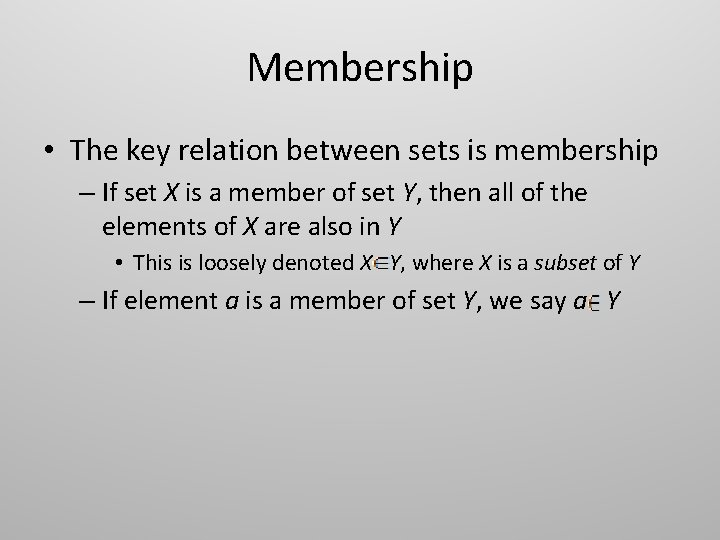Membership • The key relation between sets is membership – If set X is a member of set Y, then all of the elements of X are also in Y • This is loosely denoted X Y, where X is a subset of Y – If element a is a member of set Y, we say a Y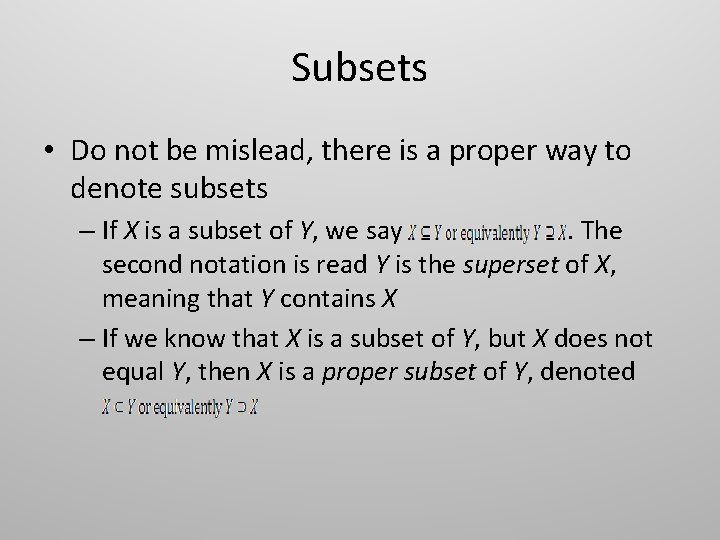Subsets • Do not be mislead, there is a proper way to denote subsets – If X is a subset of Y, we say. The second notation is read Y is the superset of X, meaning that Y contains X – If we know that X is a subset of Y, but X does not equal Y, then X is a proper subset of Y, denoted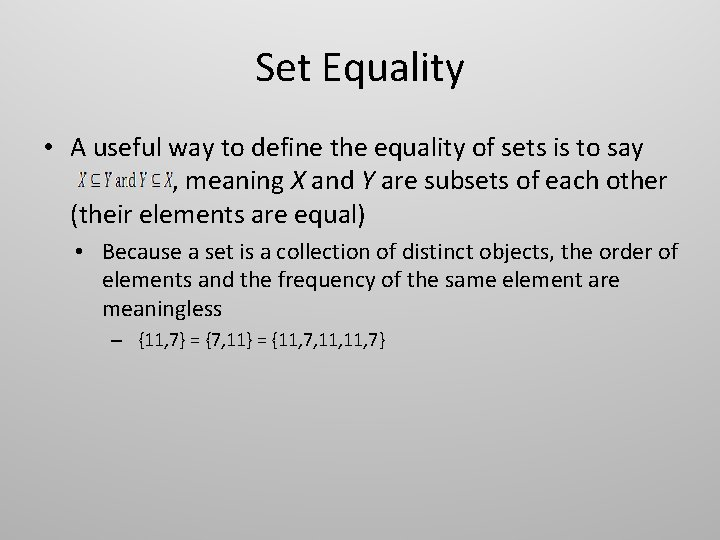Set Equality • A useful way to define the equality of sets is to say , meaning X and Y are subsets of each other (their elements are equal) • Because a set is a collection of distinct objects, the order of elements and the frequency of the same element are meaningless – {11, 7} = {7, 11} = {11, 7, 11, 7}Special Kinds of Sets • A null set (Ф)is defined as a set that is negligible in some sense – For example, if an object’s length is to be measured, the value 0 would be negligible, meaning that if an object has a length of 0, it has no length at all – An empty set is always a null set, in every situation – In general, if the value of the elements of a set can be disregarded, the set is a null set • Consider a set used to represent integers. If only integers matter, then {a, b, c} is a null set • A universal set (U) is the set that contains all meaningful objects, including itself – This set is very controversial, and in many cases is said not to exist – It is basically the opposite of the null set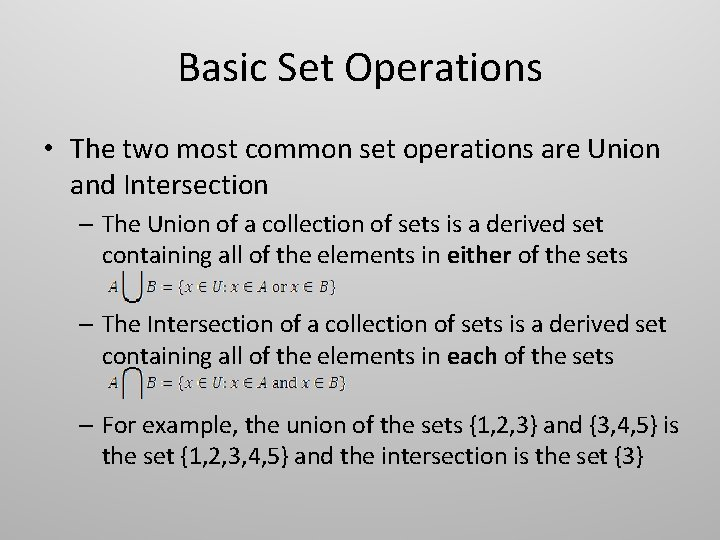Basic Set Operations • The two most common set operations are Union and Intersection – The Union of a collection of sets is a derived set containing all of the elements in either of the sets – The Intersection of a collection of sets is a derived set containing all of the elements in each of the sets – For example, the union of the sets {1, 2, 3} and {3, 4, 5} is the set {1, 2, 3, 4, 5} and the intersection is the set {3}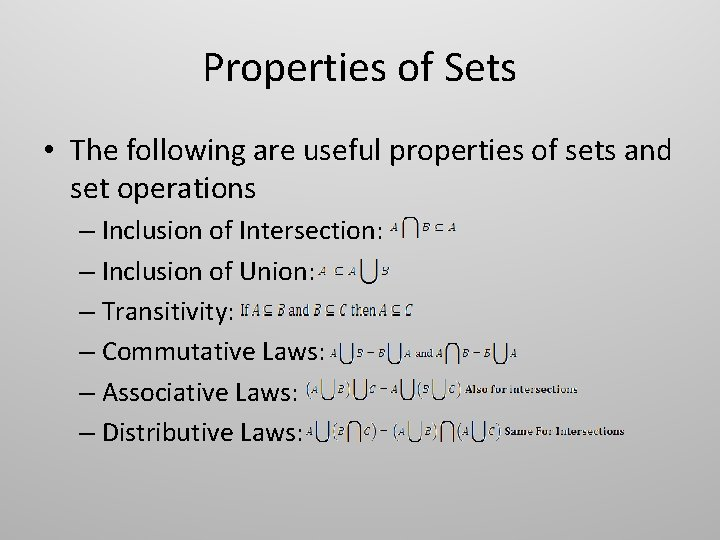Properties of Sets • The following are useful properties of sets and set operations – Inclusion of Intersection: – Inclusion of Union: – Transitivity: – Commutative Laws: – Associative Laws: – Distributive Laws: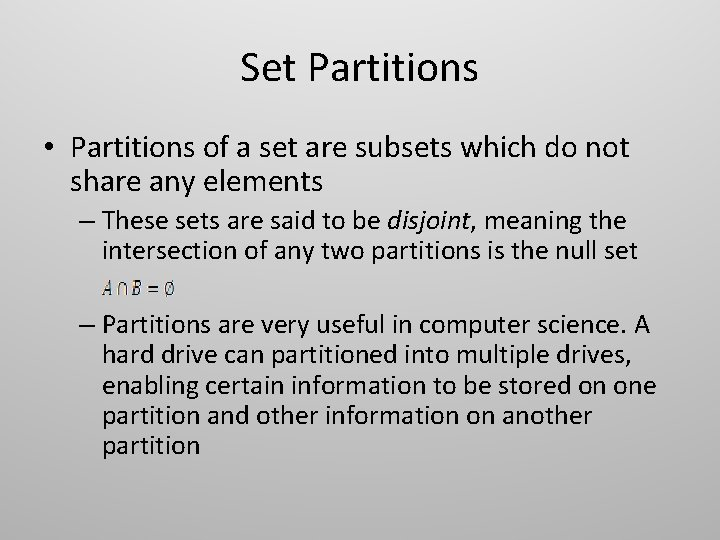Set Partitions • Partitions of a set are subsets which do not share any elements – These sets are said to be disjoint, meaning the intersection of any two partitions is the null set – Partitions are very useful in computer science. A hard drive can partitioned into multiple drives, enabling certain information to be stored on one partition and other information on another partition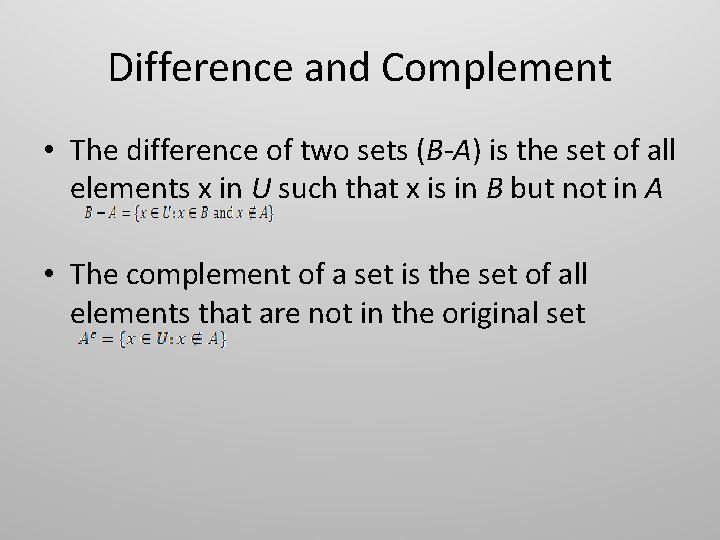Difference and Complement • The difference of two sets (B-A) is the set of all elements x in U such that x is in B but not in A • The complement of a set is the set of all elements that are not in the original setMore Set Properties – If an element is in a set’s complement, it is not in the original set – Identity Laws: – Complement Laws: – Double Complement Law: – Idempotent Laws: – Universal Bound Laws: – De. Morgan’s Laws: – Absorption Laws: – Special Set Complements: – Set Difference Law:Visual Aids • Set operations can be represented by visual aids like Venn Diagrams – Given sets A={1, 2} and B={2, 3} and outside value (4) • • • “A union B” is “A intersect B” is “A complement” is “A – B” is “Complement (A union B)” is “Complement (A intersect B)” is – Any set operation can be represented by a visual aid, regardless of complexity. This is useful in proofs!Power Sets • The power set of set A is the set of all subsets of A – Consider the set {1, 2} • The power set of {1, 2} contains all subsets, so the null set and the original set itself are also elements • This is denoted • It has been proven (through induction) that a set of n elements has exactly subsetsTuples • A set, such as {1, 2} is equal to all other sets that contain only the elements {1, 2} in any order and frequency, such as {1, 2, 1, 1} • The opposite is true of an ordered tuple – The ordered tuple (x, x, …, x[n]) is equal to the ordered tuple (y, y, …, y[n]) iff “x = y, x = y, …, x[n] = y[n]” • Each element must equal its partner – (a, b) = (c, d) iff “a = c and b = d” – An ordered 2 -tuple is denoted ((x, y), …, (x[n], y[n])) and is called an ordered pair. A 3 -tuple is an ordered triple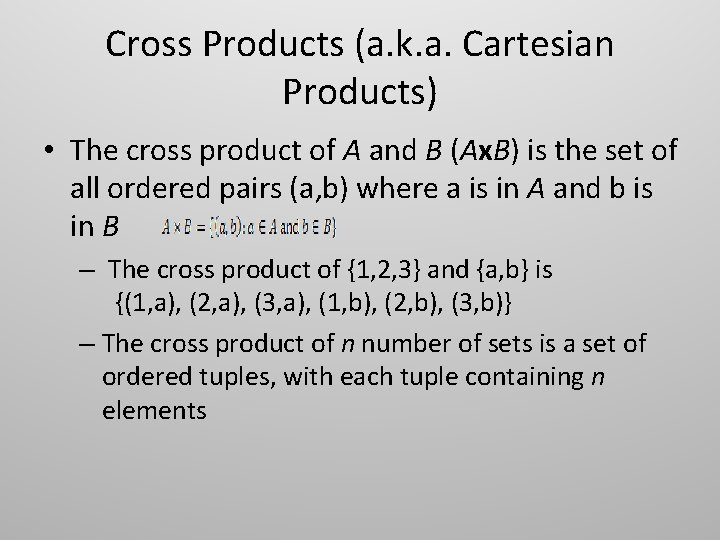Cross Products (a. k. a. Cartesian Products) • The cross product of A and B (Ax. B) is the set of all ordered pairs (a, b) where a is in A and b is in B – The cross product of {1, 2, 3} and {a, b} is {(1, a), (2, a), (3, a), (1, b), (2, b), (3, b)} – The cross product of n number of sets is a set of ordered tuples, with each tuple containing n elements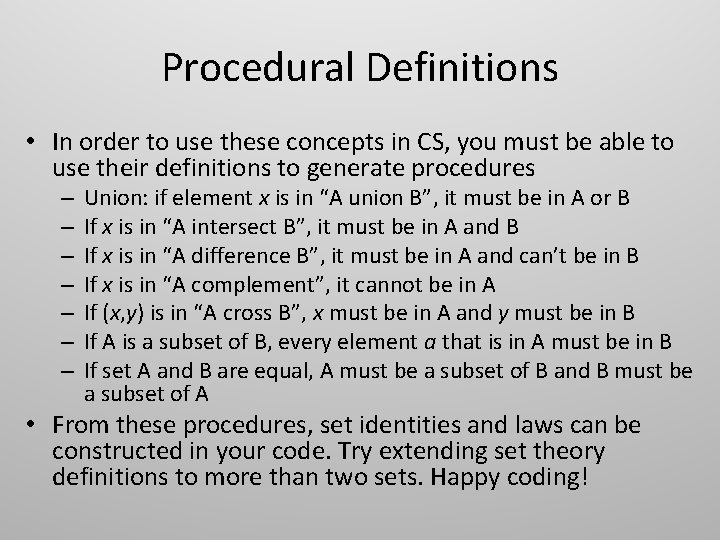Procedural Definitions • In order to use these concepts in CS, you must be able to use their definitions to generate procedures – – – – Union: if element x is in “A union B”, it must be in A or B If x is in “A intersect B”, it must be in A and B If x is in “A difference B”, it must be in A and can’t be in B If x is in “A complement”, it cannot be in A If (x, y) is in “A cross B”, x must be in A and y must be in B If A is a subset of B, every element a that is in A must be in B If set A and B are equal, A must be a subset of B and B must be a subset of A • From these procedures, set identities and laws can be constructed in your code. Try extending set theory definitions to more than two sets. Happy coding!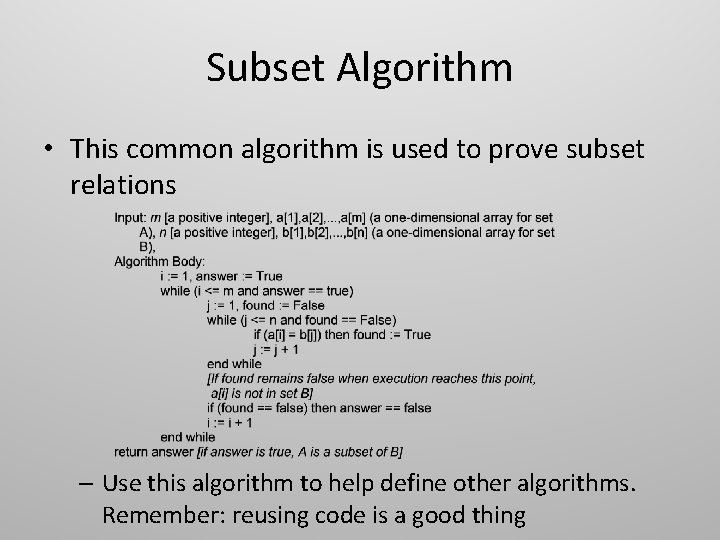Subset Algorithm • This common algorithm is used to prove subset relations – Use this algorithm to help define other algorithms. Remember: reusing code is a good thing